《从问题到程序：用Python学编程和计算》——3.2　递归

+关注继续查看

3.2.1　递归定义的函数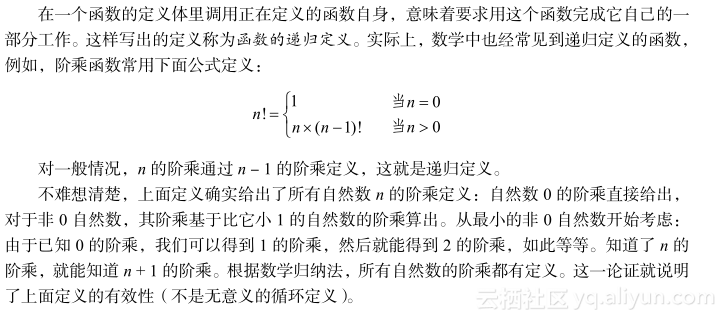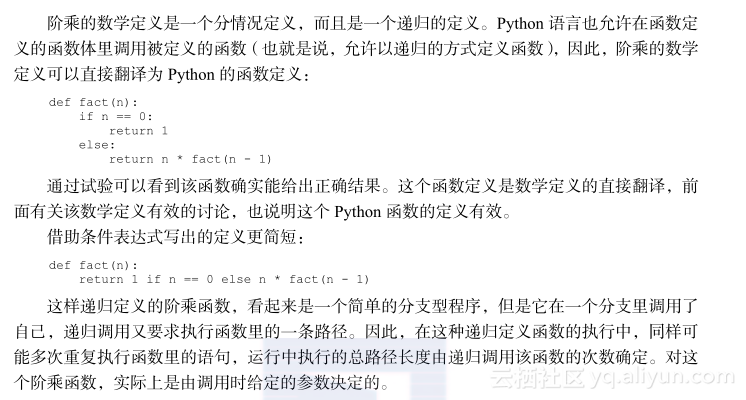3.2.2　乘幂的计算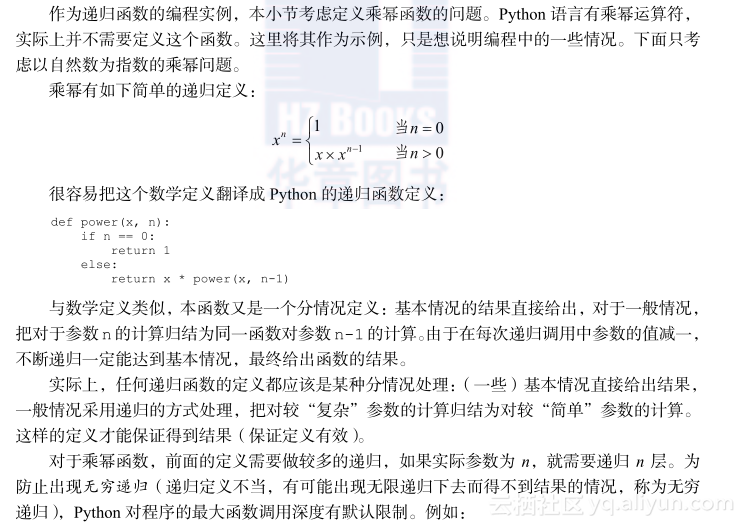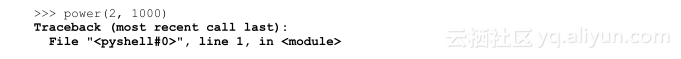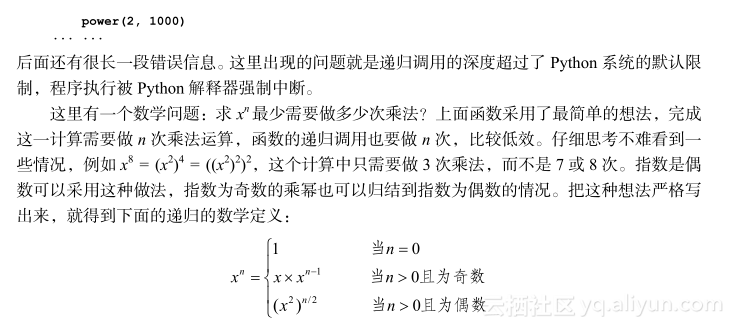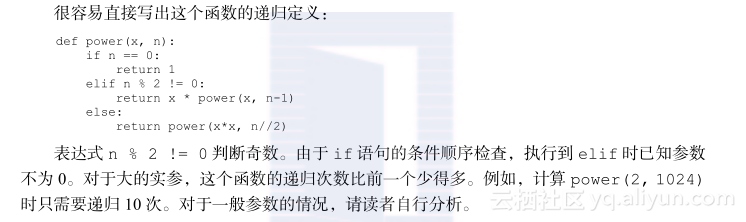3.2.3　循环和递归

def power(x, n):
p = 1
while n > 0:
if n % 2 != 0:
p *= x
n -= 1
else:
x *= x
n //= 2
return p

p是这里的累积变量，循环中把x的一些幂次累积到p上，最终得到x的n次幂。循环里区分了几种情况：指数n等于0时累积工作完成，循环结束，变量p的值即为所需。循环体就是一个if语句，两个分支分别处理指数为偶数和奇数的情况，其中用了几个扩展的赋值语句更新变量。注意，对p的初始化保证，即使这个循环的体一次也没有执行，得到的结果也是对的。

3.2.4　斐波那契数列的计算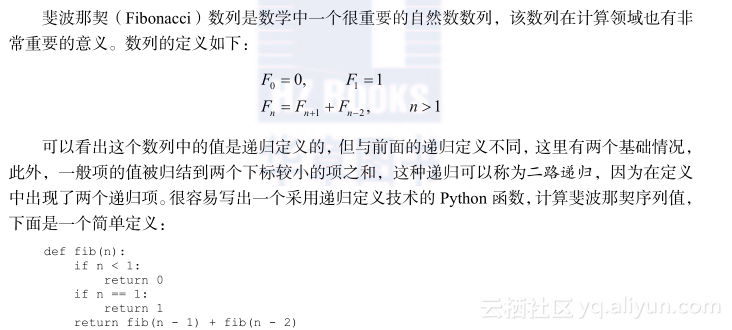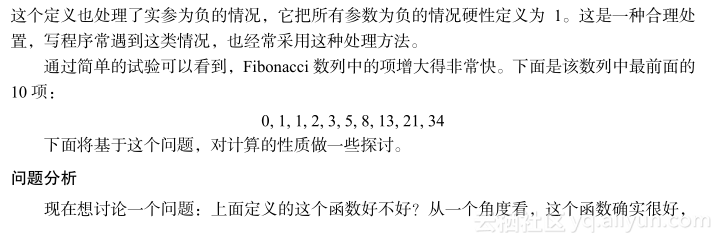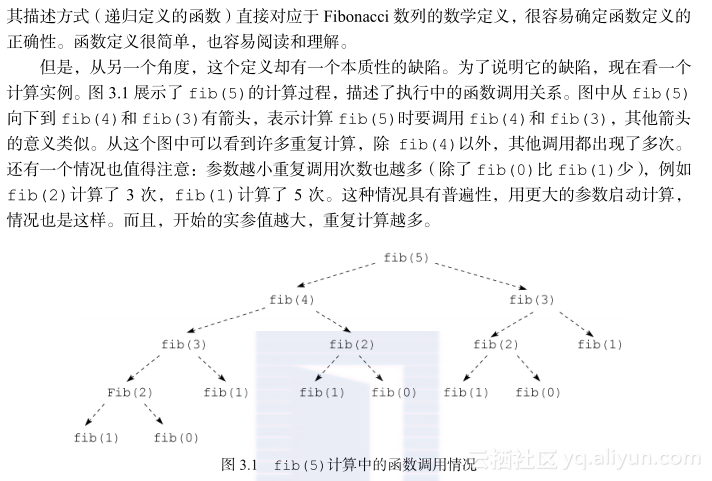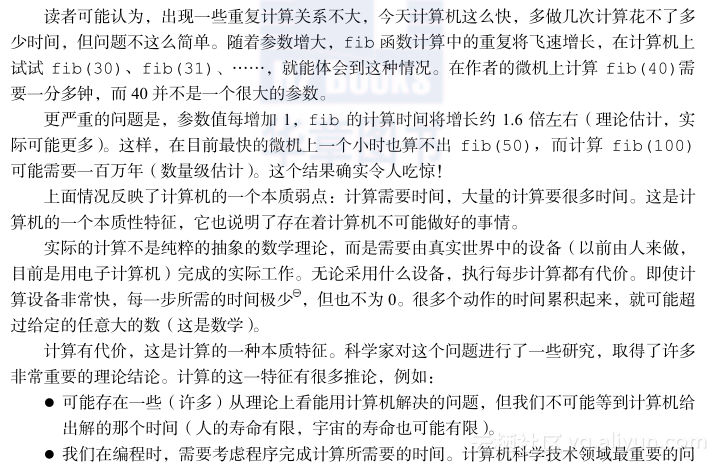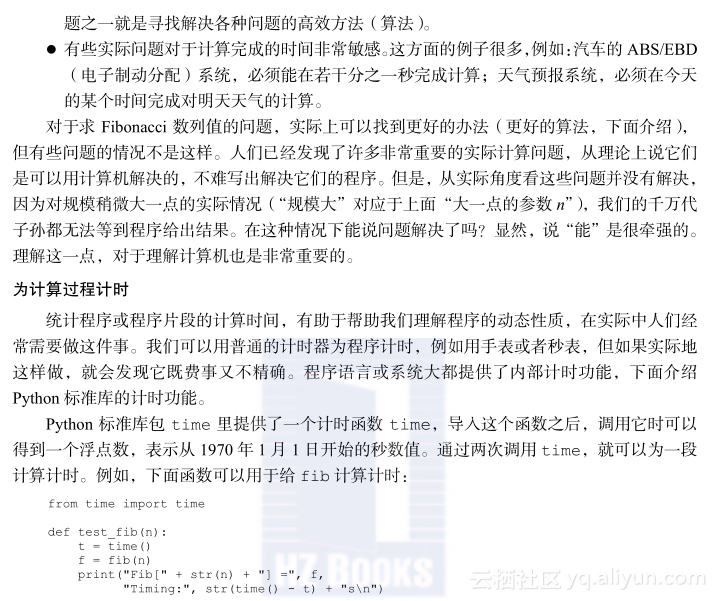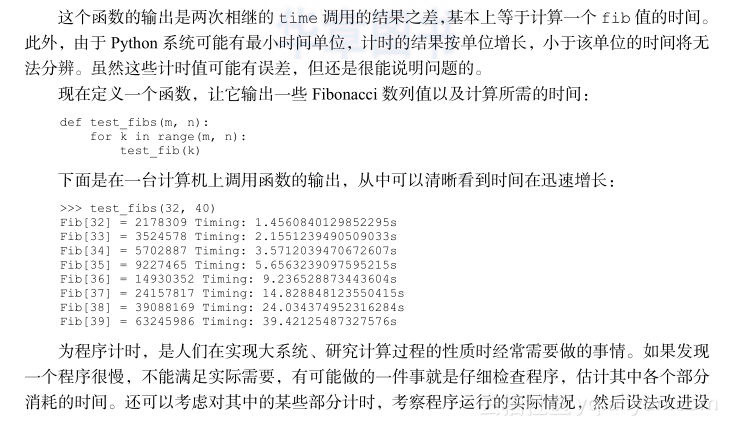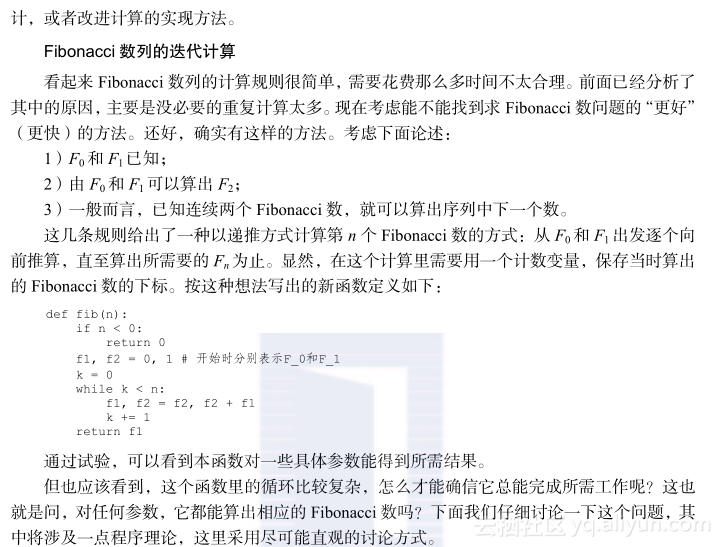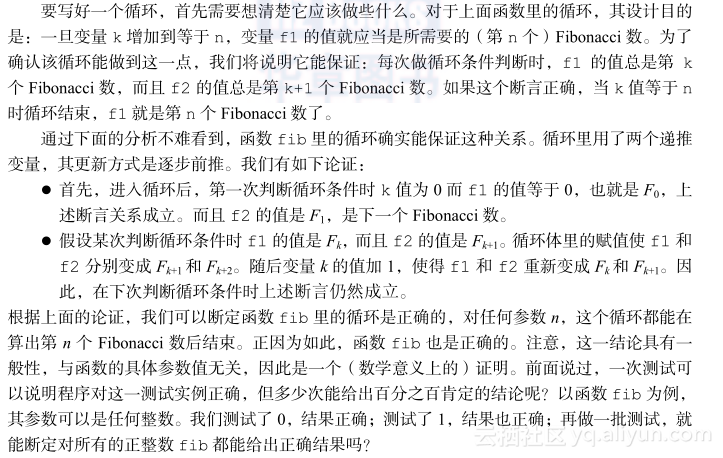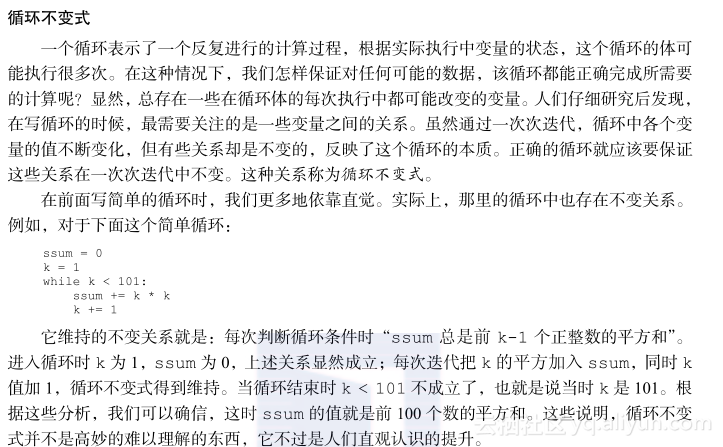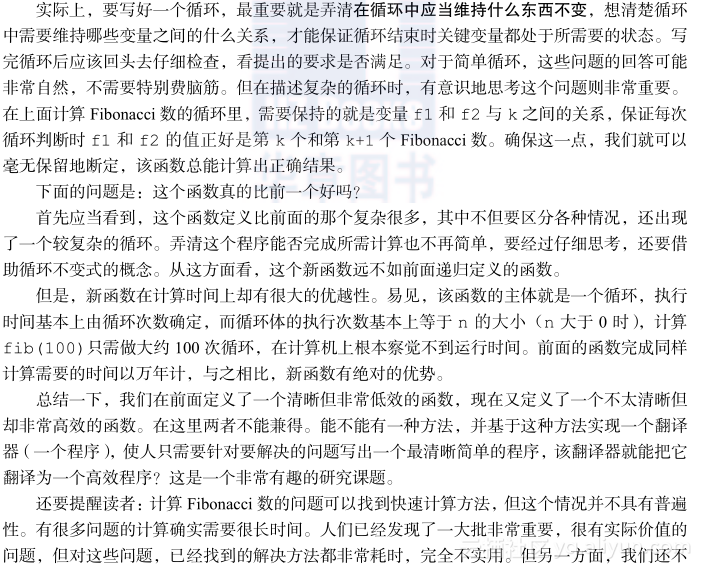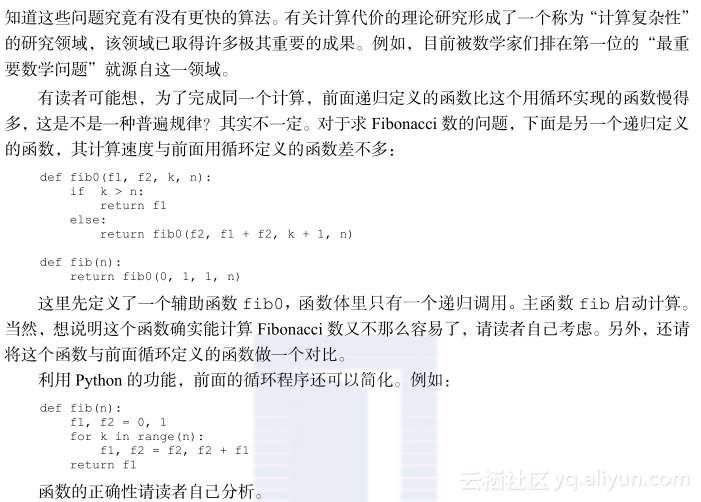朴素解法

if m % k == 0 and n % k == 0:
d = k;  # k是新的公约数，而且更大

def gcd (m, n):
d = 1
k = 2
while k <= m and k <= n:
if m % k == 0 and n % k == 0:
d = k
k += 1
return d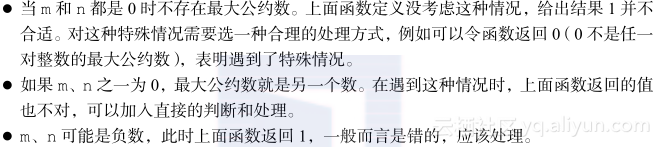if m == 0:
return n
if n == 0:
return m
if m < 0:
m = -m
if n < 0:
n = -n

辗转相除法def gcd(m, n):
if n % m == 0:
return m
return gcd(n % m, m)

def gcd_main(m, n):
if m < 0:
m = -m
if n < 0:
n = -n
if m == 0:
return n
return gcd(m, n)

1）循环开始时，m和n是求最大公约数的出发点。

2）每次循环判断n % m是否为0。如果为0，m就是最大公约数，结束。

3）否则做变量更新：更新后的n取原来m的值，而m取原来n % m的值。这两个变量更新可以用一个平行赋值实现：

m, n = n % m, m

def gcd(m, n):
if m == 0:
return n
while n % m != 0:
m, n = n % m, m
return m

n的值为0以及m和n的值都为0的情况没有特殊处理，因为这些处理都已经包含在定义里了。读者很容易弄清楚这一点。上面函数里也有一个较复杂的循环。按照前面说法，一个循环中总要维持某种不变关系，以保证循环的正确性。那么，上面函数里的这个循环维持的是变量之间的什么关系呢？这一点也请读者考虑。

3.2.6　不容易用循环求解的递归问题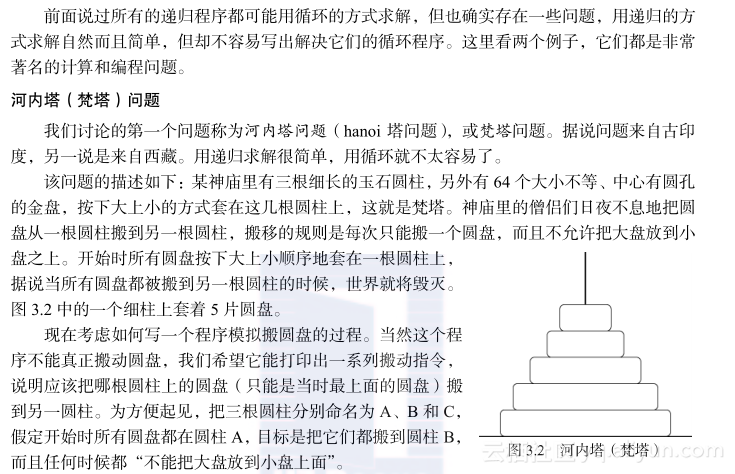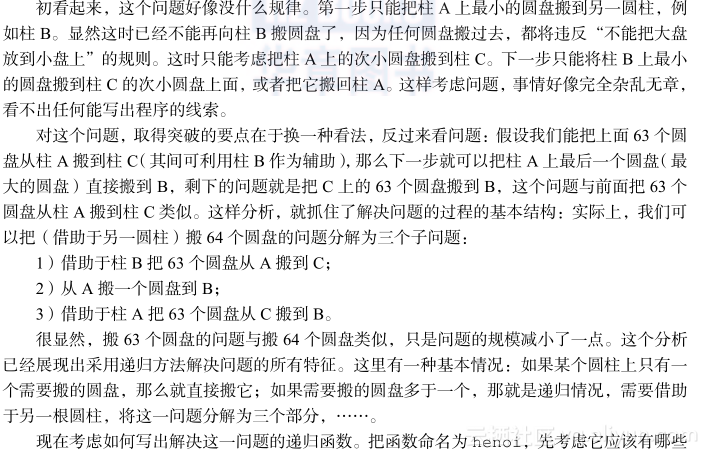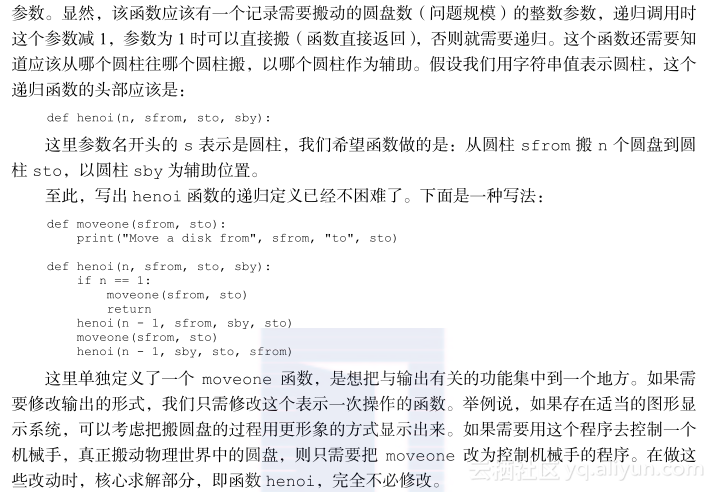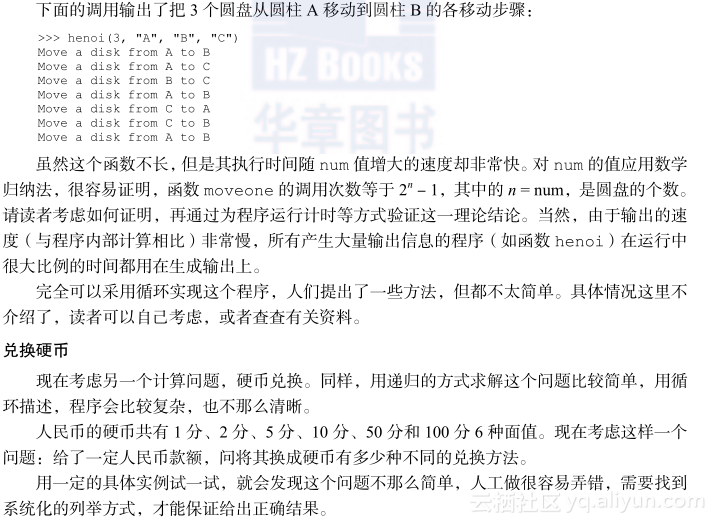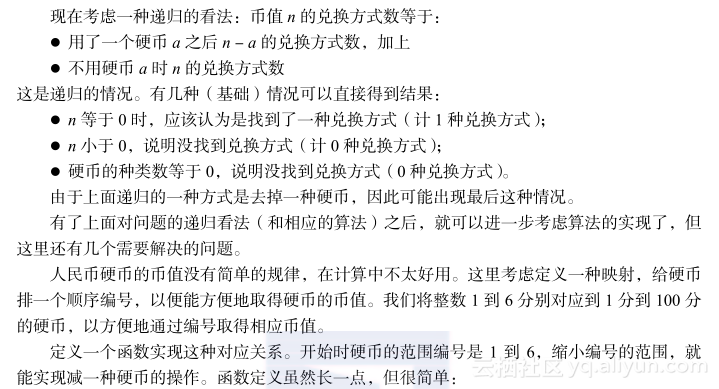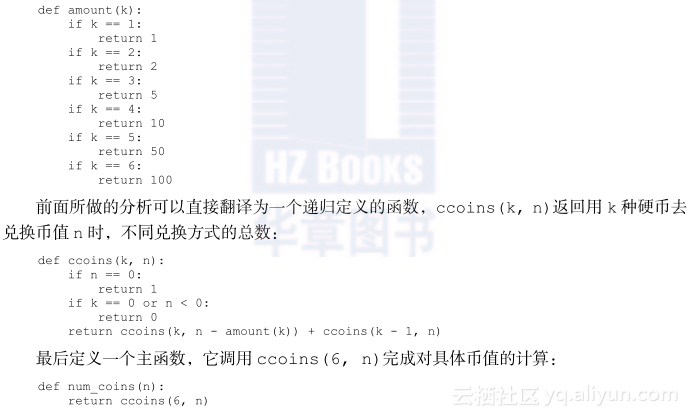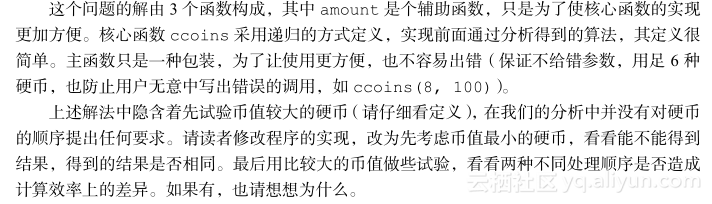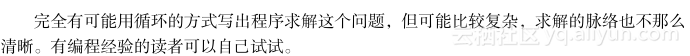3.2.7　更复杂的递归情况

def even(n):
if n == 0:
return True
else:
return odd(n-1)

#even(4) # 在这里调用将会报销

def odd(n):
if n == 0:
return False
else:
return even(n-1)《从问题到程序：用Python学编程和计算》——2.10　若干Python机制及其他
905 0《C语言程序设计》一3.4　案例学习——计算一年中某月份的总天数

1113 0《并行计算的编程模型》一3.6.3　锁

725 0《从问题到程序：用Python学编程和计算》——2.11　补充材料
1743 0【转】C# 计算程序运行时间
1 //计算程序运行时间(.net1.1 于.net2.0的区别)在.net2.0中提供了Stopwatch类，简单例子  2 using System.Diagnostics;  3  4 private Stopwatch stw = new Stopwatch();  5  6 pri...
600 0《并行计算的编程模型》一3.7　集合操作

783 0《并行计算的编程模型》一3.6.4　wait和wait_until

844 0《Python高性能编程》——2.3　计算完整的Julia集合
3159 0Python编程：10个面试常问的问题
Python编程：10个面试常问的问题
85 0《从问题到程序：用Python学编程和计算》——练习
5135 0
10059

0

+ 订阅

《2021云上架构与运维峰会演讲合集》

《零基础CSS入门教程》

《零基础HTML入门教程》